Next: A.2 Longitudinal Field Polarization Up: A Muonium Spin Polarization Previous: A Muonium Spin Polarization

# A.1 Transverse Field

Now that we have the eigenvectors and eigenvalues of all four muonium states it is a straightforward task to calculate the matrix elements of the muon spin operator. Initially, the muon beam is virtually 100% spin polarized in any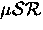experiment using surface muons. In the case of a transverse field experiment the initial muon spin direction is perpendicular to the applied magnetic field, conventionally along the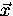axis, so we have an initial muon state given by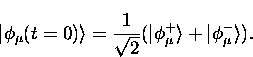(13)
Since electrons of the stopping medium (which are captured by muons to form muonium) are unpolarized, we have two initial muonium states possible - with the electron spin either up or down. It is has always been assumed that these are formed with equal probability; no observable departure from this has ever been reported, so we have equal contributions from each of the initial states

Considering the first of these we can write, using Eq. (A.17),

At a later time t, when the initial state has evolved under the Hamiltonian, we have

Expanding the Hamiltonian in terms of operators and the wavefunctions in terms of the basis states, we have

giving

So we have, for that fraction of muonium formed with electron spin up'', the contribution to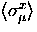of

The other fraction, with an initial state in which the electron spin is down",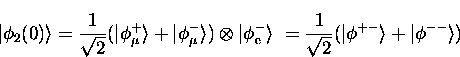(14)
is treated in a similar way, giving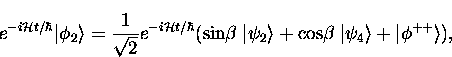(15)

and a contribution to the spin polarization of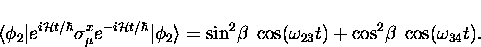(16)
The total polarization is simply the sum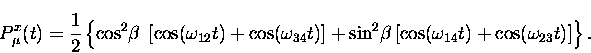(17)

In weak magnetic fields of not more than about 8 G the difference between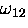and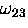is small enough that it can usually be ignored; the signal appears to have only one component at the average muonium frequency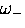.In higher field the splitting that originates with the hyperfine interaction term of the Hamiltonian is immediately apparent in the polarization function as beating of the these two components, the hyperfine frequency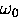can be obtained from the difference of the two frequencies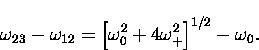(18)
For unperturbed muonium (in vacuum, for example) the hyperfine frequency is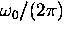= 4463 Mhz.  (In condensed matter nearby molecules can cause a distortion of the 1s orbital of the muonium electron, changing the electron spin density at the muon which is reflected in a shift of the hyperfine frequency.)

Since the high frequency transitions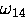and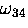are not resolved by the timing electronics of mostspectometers, those terms average to zero, and in fields sufficiently weak that the amplitudes of the remaining signals are approximately equal, the polarization function reduces to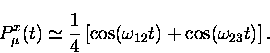(19)Next: A.2 Longitudinal Field Polarization Up: A Muonium Spin Polarization Previous: A Muonium Spin Polarization